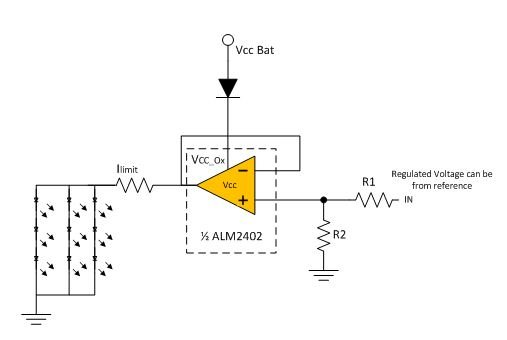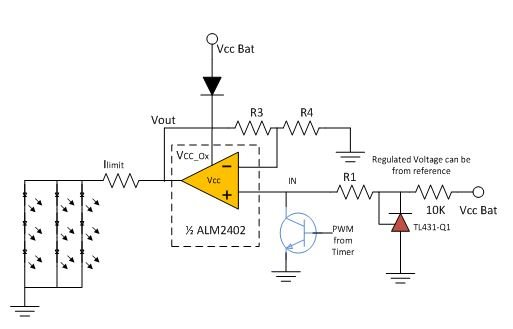# Using dual high-current op amps to drive automotive LED lights

Automotive applications require that output Light Emitting Diode (LED) be driven by a constant current source with short-circuit protection for the LED driver. This current solution is a more discrete approach to the problem, with amplifier and comparators to drive field-effect transistors (FETs) or integrated protection FETs. This enables the circuit to have protection, but at a higher cost and increased board space.

The high-current capability of TI’s ALM2402, a dual high-voltage, high-current operational amplifier (op amp) enables it to drive loads up to 400mA; applications include lighting automotive tail lights or direction indicators. The ALM2402 has short-to-battery and short-to-ground protection and also features a flag output that enables the control system to shut down the device in case of adverse conditions. In this post, I will explain how to use the ALM2402 in LED automotive applications such as daytime running lights, brake lights and direction indicators.

First, consider the following two items:

• You have to regulate VLED at the output.
• Current has to be regulated at the output.

Figure 1 shows an implementation of the regulated high-side drive for an LED taillight application. The diode in the series is required to protect against reverse battery conditions in the harsh working environment.Figure 1: LED drive using the ALM2402-Q1

Let us consider a design scenario where VccBat = 12Vand Vled = 2.6V. Let’s assume not. of LED in series = 3, there are 3 parallel strings of these LEDs. Max current through each LED = 30mA.These conditions require you to design for a maximum current of 90mA, since three LED strings are in parallel. The total voltage across LEDs = 7.8V. You can regulate the Vout of the ALM2402 to ensure constant current across LEDs by adjusting R1 and R2. In this case, suppose you have IN =12V (from a battery or a regulated switched-mode power supply [SMPS]). Assuming that R1 =100KΩ, R2 is calculable using Equation 1:

Vout = 12*R2/(R1+R2)                   (1)

To ensure the proper operation of LEDs with regulated current, you can regulate the output of ALM2402 to 9V. The choice of 9V ensures regulated voltage across LEDs with Vbat fluctuations.

Considering that Vout = 9, R2 = 300KΩ.  (2)

Note there is an I limit current-limiting resistor in series with LED’s. This resistor basically sets the total current through the LEDs. The equation to calculate the series resistance is shown below:

R I limit = (Vled*No. of LED in series-9V)/(90mA) =13.3Ω                               (3)

Pulling IN, which is non inverting input for ALM2402, down can disable the output as shown in figure 2. This allows the circuit to work as a turn/direction indicator application in automotive as well. You can use a timer IC like the NE555 to control the flash rate.Figure 2: Examples with reference voltage at IN

You can also use a precise reference like the TL431-Q1 for output voltage control. The output voltage is based on this reference voltage resulting in precise output control.  In this example The TL431-Q1 provides a reference of 2.5V. In the case of Figure 2, vout can be designed using below equation.

Vout = 2.5 X (R3/R4 +1)                 (4)

If we choose R3 = 200KΩ, R4 is calculated to be close to 77KΩ. You can adjust the I limit resistor to compensate for the long wires from the control board to the LED boards to ensure precise LED currents.

ALM2402-Q1  is a dual amplifier in single package allowing it to be a cost and performance optimized solution to driving LED’s in automotive applications. Driving LEDs in linear mode is simple but does have challenges around designing the layout for proper thermal dissipation. For more efficient power conversion, it is advised to use switch mode power supplies. For more information about driving your LEDs, see resources on our website.